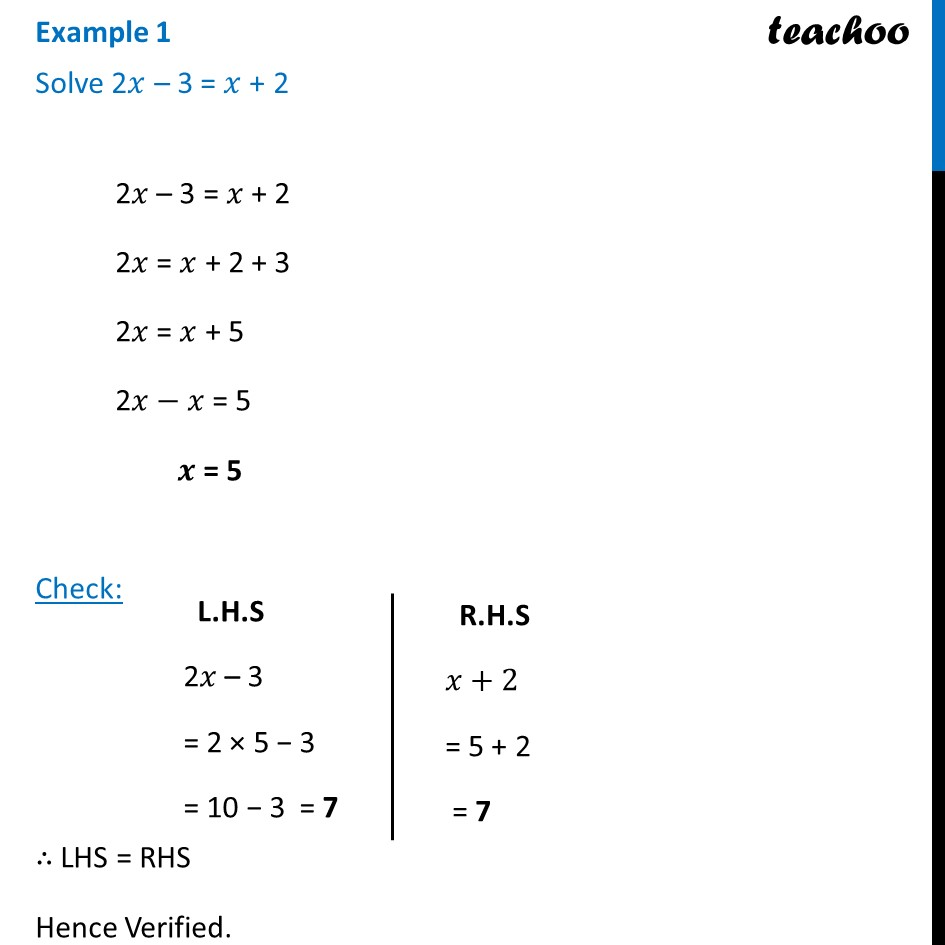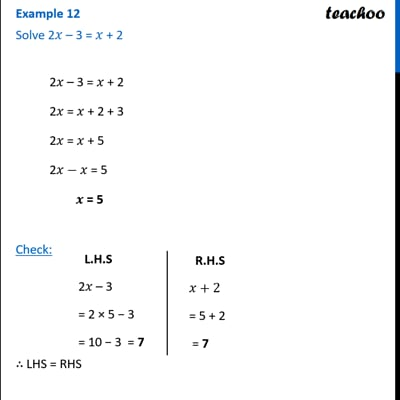Examples

Chapter 2 Class 8 Linear Equations in One Variable
Serial order wiseThis video is only available for Teachoo black users

Learn in your speed, with individual attention - Teachoo Maths 1-on-1 Class

### Transcript

Example 1 Solve 2𝑥 – 3 = 𝑥 + 22𝑥 – 3 = 𝑥 + 2 2𝑥 = 𝑥 + 2 + 3 2𝑥 = 𝑥 + 5 2𝑥−𝑥 = 5 𝒙 = 5 Check: ∴ LHS = RHS Hence Verified. L.H.S 2𝑥 – 3 = 2 × 5 − 3 = 10 − 3 = 7 R.H.S 𝑥+2 = 5 + 2 = 7